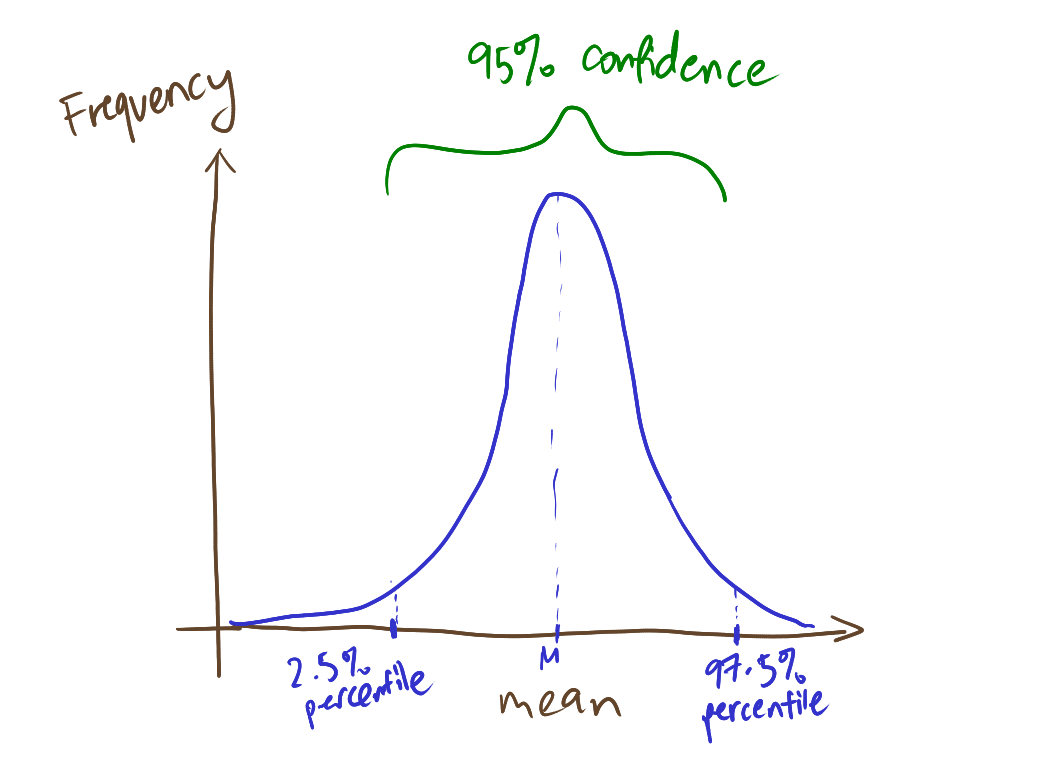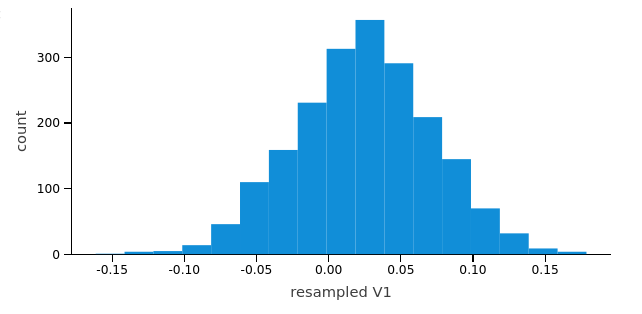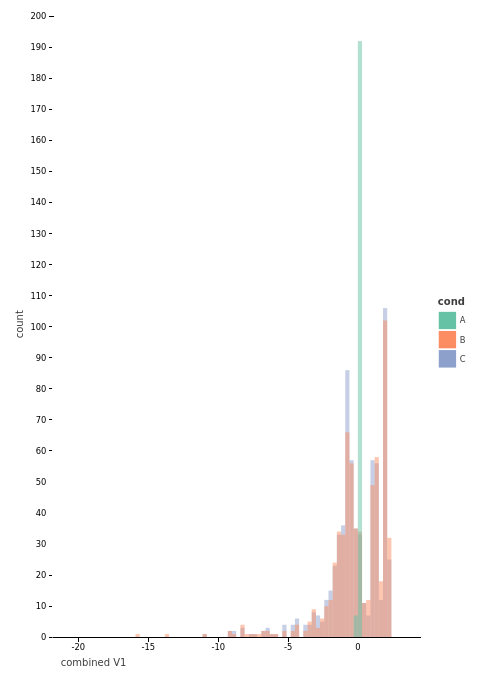So far, we have generated random numbers based on the assumption that we know the probabilities of the numbers we are generating. But what if, we have a dataset that we are not sure what “standard “type” of distribution is matches, but need to generate random numbers from it.

What about using inverse transform sampling? Well, recall that ITS requires us to have knowledge about a distribution’s cumulative distributive function. Without knowledge of the distribution’s type, it will be more difficult to deduce the cumulative distribution function as we need to add up the individual probabilities at various points of the distribution

## Sampling with an empirical method

What’s empirical?

This means that we will have to draw conclusions or patterns based on the sample distribution presented to us.

The empirical distribution function is one such method, where we compute the sample’s distribution function from sampling.

Distribution function? Recall earlier that we have learnt about the cumulative distribution function. It is a form of “step function”, that steps up in interval by 1/n at each of the n data points.

The value of the empirical distribution function at a given point$x$ is obtained by:

• adding up the no. of observations that are$\le x$
• dividing the number by the total number of observations$n$, thus obtaining the percentage of observations$\le x$

This can be expressed as$\frac{1}{n} \sum_{i=1}^n \mathbf{1}_{X_i \le t}$

where

•$\mathbf{1}_{X_i \le t}$ is sort of a boolean “operator” which reflects if the continuously sampled value is equal to 1 if it is less than x (the current sample) and 0

Hence, we can think of the empirical distribution function as “implementing” the cumulative distribution function

First, let us see how the empirical distribution function is used.

				
%use s2

// generate some samples from standard normal distribution
var uniform = MersenneTwister()
uniform.seed(1234567890L)

var rng1 = Zignor2005(uniform) // mean = 0, stdev = 1
var N:Int = 200
var x1 = DoubleArray(N)

for (i in (0..N-1)){
x1[i] = rng1.nextDouble()
}

// empirical distribution function helps create a cumulative distribution function
var dist2 = EmpiricalDistribution(x1)

var rng2 = InverseTransformSampling(dist2)

var x2 = DoubleArray(N)

for (i in (0..N-1)){
x2[i] = rng2.nextDouble()
}



We use the Mersenne Twister to generate a set of random numbers.

We then make use of Inverse Transform Sampling (ITS) to generate the corresponding sample, as the Cumulative Distribution function (CDF) has already been “generated” by the empirical distribution function.

				
// check properties of random variates
var varianc = Variance(x2)
val mean = varianc.mean()
val stdev = varianc.standardDeviation()

println("mean = %f, standard deviation = %f".format(mean, stdev))

//check if the samples are normally distributed
var test = ShapiroWilk(x2)
println("ShapiroWilk statistics = %f, pValue = %f".format(test.statistics(), test.pValue()))


				
mean = -0.018069, standard deviation = 0.961254
ShapiroWilk statistics = 0.982637, pValue = 0.014264



ShapiroWilk is a type of statistical test that tests if something is a normal distribution. We can see that the resulting pValue is 0.01 which is not small enough to reject the null hypothesis that the distribution is normal. Thus, we can conclude that the RNG based on the empirical method is close enough to the original.

Notice though that the statistics are remarkably close to the “ideal” mean of 0 and standard deviation of 1.

### Re-sampling Method: Another way to deduce a distribution from datasets

Continuing with our earlier example about credit card fraud, let’s take a look at a real-life dataset.

				
//Data Wrangling library https://github.com/holgerbrandl/krangl
%use krangl

// read the CSV file from the web into a data frame

// print out the first 5 rows of the dataset


Time
V1
V28
Amount
Class
0
-1.3598071336738
-0.0210530534538215
149.62
‘0’
0
1.19185711131486
0.0147241691924927
2.69
‘0’
1
-1.35835406159823
378.66
‘0’

This is a large dataset! There are many columns, notably V1 to V28 which are “PCA (Principal Component Analysis) transformed”

If we take a look at the dataset, “class” stands out as it is one of the columns as it indicates if the row is a fraudulent credit card transaction. We can create a sub dataframe consisting of only this column.

				
val classvals = df.groupBy("Class")
classvals.count()


Class
n
‘0’
284315
‘1’
492

Notice something? The number of no-fraud cases vastly outstrip that of the transactions that contain frauds!$\frac{492}{(284315+492)} \times 100 \approx 0.17\%$

Phew, there are only about 0.17% of the dataset forming the fraud cases!

But, wait!  This dataset is highly unbalanced. This is especially a problem for many ML algorithms as they do not consider the difference in distribution of classes.

To address this issue, methods include resampling (oversampling the minority, under-sampling the majority, generating “new” samples from the minority).

However, do note that this is highly dependent on the algorithms used, not to mention whether the initial sample reflects the real-world scenario!A confidence interval is a way of describing the certainty of whether random samples taken follow the test statistics (eg. mean) given

Resampling is a broad term to refer to a variety of methods for doing one of the following:

• Creating more accurate samples of a distribution (through replacement) & determine confidence intervals (bootstrapping)
• Sampling “without replacement” to test hypothesis with an aim where a sample taken has no effect on the rest of the distribution (Permutation methods)
• Where populations have unique “known” properties, we can also continuously sampling an observe how different sampling methods affect these properties (Monte Carlo Methods)

### What is Bootstrapping?

💡 The main idea is to deduce the distribution through only sampling of the distribution. There are 3 main ways ( re-sampling [aka non-parametric], “adding noise” [aka semi-parametric] and simulation [“parametric”] ). We will be focusing on the Re-sampling bootstrap method.

Important Things to Note:

• Unlike other samples, a resampled bootstrap sample contains the same number of samples as the distribution size. For this to happen, data is taken with replacement.
• This allows the resampled data to have a remarkably similar replicated distribution compared to other methods such as semi-parametric ones.

Now, let us implement the resampling in Kotlin. First, we extract the column (“V1”) of values from the data frame, and insert it into an array (known as “sample”)

We run this code in-line with the previous one, so that the variable df is carried forward

				
%use s2

val size:Int = df.get("V1").values().count()
var sample = DoubleArray(size)
val v1_data = df.get("V1").values()

for (i in (0..size-1)){
sample[i] = v1_data[i].toString().toDouble()
}



After creating the sample, we can conduct resampling. Each resampling gives a re-sampled array of data. We can then deduce the mean from this try, and repeat the re-sampling 500 times, to get a “distribution” of means of the resample.

				
var boot = CaseResamplingReplacement(sample)
boot.seed(1234567890L)

val B:Int = 500
var means = DoubleArray(B)

for (i in (0..B-1)){
var resample = boot.newResample()
means[i] = Mean(resample).value()
}

var mean = Mean(means).value()
var varianc = Variance(means).value()

println("mean = %f, variance of the estimated mean = %f".format(mean,varianc))


				
mean = -0.000011, variance of the estimated mean = 0.000012




We can compare this mean to the original distribution with df.get("V1").mean(). How close is it?

To get a better idea of how the original distribution looks like, let us visualise the original plot. We will be using a random number generator for this to create a sample.

				
val size:Int = 2000
var sample = DoubleArray(size)

val v1_data = df.get("V1").values()

for (i in (0..size-1)){

var temp:Double = v1_data[(0..284316).random()].toString().toDouble()
sample[i] = temp

}

val values = mapOf&lt;String, List&gt;(
"V1" to sample.toList(),
)

val plot = lets_plot(values) { x = "V1" } + // supply data; set legends
ggsize(500, 250) + // plot size
geom_histogram(binWidth=0.5, bins=200)   // draw a basic histogram
plotWe can then visualize the Resampled version.

				
var boot = CaseResamplingReplacement(sample)
boot.seed(1234567890L)

val B:Int = 2000
var means = DoubleArray(B)

for (i in (0..B-1)){
var resample = boot.newResample()
means[i] = Mean(resample).value()
//println(resample)
}

val values2 = mapOf&lt;String, List&gt;(
"resampled V1" to means.toList()
)

val plot = lets_plot(values2) { x = "resampled V1" } + // supply data; set legends
ggsize(500, 250) + // plot size
geom_histogram(binWidth=0.02, bins=200)   // draw a basic histogram
plotNotice how much “tighter” the distribution is! It closely resembles the shape of the gaussian or “normal distribution”, a product of the central limit theorem.

As you can see, the histogram provides an estimate of the shape of the distribution of the sample mean, which we can then use to answer questions about how the mean varies across different re-samples.

Now, let us compare the one-instance of the re-sampled version, the means of the resampled versions, as well as the original distribution by overlaying their histograms on top of each other:

				
val values2 = mapOf(
"cond" to List(B) { "A" } + List(B) { "B" } + List(B) { "C" },
"combined V1" to means.toList()+sample.toList()+boot.newResample().toList()
)

val plot = lets_plot(values2) { x = "combined V1"; fill="cond" } + // supply data; set legends
ggsize(600, 600) + // plot size
geom_histogram(binWidth=0.1, bins=200, alpha=0.5, position=Pos.identity)  // draw a basic histogram

plot• A: Mean of Resampling
• B: “Original”
• C: Single Resample

Notice how the mean is much sharper when viewed this way!

This is because in order to fit all 3 graphs in one, we had to reduce the binWidth, and more values were “bundled together”, creating a much sharper peak.

Also notice how one-instance of the re-sampled version is fairly close to that of the original distribution, yet for values closer to the mean, the resample tends to have higher values than the original.